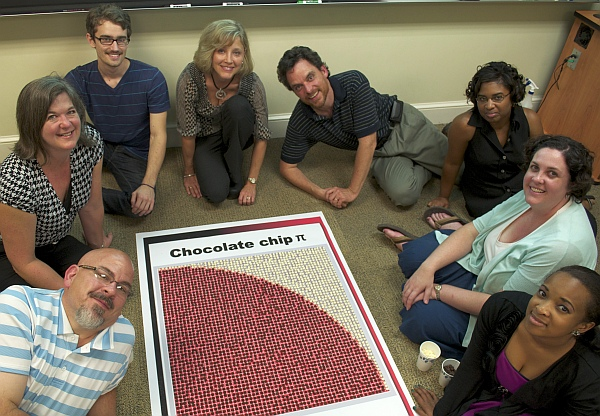By George Hart for the Museum of MathematicsThere are many ways to calculate an approximation to pi, but rarely is math as delicious as in this idea from Davidson College professor Tim Chartier. Make a quarter circle in a square of graph paper and place chocolate chips on the squares that lie completely inside the circle. If you now count the chips and compute four times the number of chocolate chips divided by the total number of squares, that will be approximately pi. Here, there are 22 chips out of 36 squares, so we calculate 4∙22/36=2.444, which is off by about 0.7 from 3.14, so is not a very close estimate of pi, but we can improve it.But if you are willing to invest in more chocolate, you will get a better answer. In the 11×11 grid below, you can count 83 chips, giving a somewhat better approximation of 4∙83/121=2.74.For an even better approximation, you’ll want to have a group of helpers. Tim and friends counted 2232 chips in a 54×54 grid, resulting in an approximation of 3.06, which is getting pretty close to pi. The best thing about this chocolate calculation is that it is sure to end with a pi eating event. The full report is here.More:
See all of George Hart’s Math Monday columns

## 16 thoughts on “Math Monday: Candy Pi Calculator”

1.Anonymous says:

not quite as sweet… but one could just cut along the circle line and use the proportion of the weights of the paper  (eating chocolate the whole time)

2.Cory Poole says:

I’ve always like using Buffon’s needle problem in my Calculus classes.  Hmmm….adopting it to use candy would make that better though!

3.Andrew Clayton says:

Faster approach to the correct result: add some caramel chips into the mix. Use chocolate for entirely within the circle, white for entirely outside the circle, and caramel for squares on the line. Average  chocolate and chocolate + caramel and then divide by the total and multiply by 4. Doing this gets you pi=3.06 in the first example and pi=3.09 in the second. I’m not counting it out for the third. :)

4.Egon Geerardyn says:

Like Andrew Clayton, I’d like to suggest a faster approximation for pi. How to do it: put a black chocolate on every square that is mostly (or completely) colored and put white chocolates on every other square. If you can’t decide whether it’s mostly colored or not, just pick one at random. A bit harder perhaps, but it yields 3.11 and 3.14 for the first two cases, so you get immediate results.

1.Jason Hooker says:

I’m glad I’m not the only one who thought of this solution. It yields much more accurate results than the underestimation provided by the original method.

5.John Martell says:

Well some of the chocolate chips should be replaced with white ones, looking at where most of the square is.

Can get 3.11 for the first graph if you defect the top left 2, bottom right 2 and 45deg 2; these are mostly under the curve.

So… it is even closer than shown in article calcs

6.Anonymous says:

Weird way to measure pi. Pi is defined as the ratio of the circumference to the diameter. It would be more efficient to use the chips as a unit. Line up chips around the curve and along the radius. Pi would then be calculated by (circumference chips x 4)/(radius chips x 2).

7.Anonymous says:

Weird way to measure pi. Pi is defined as the ratio of the circumference to the diameter. It would be more efficient to use the chips as a unit. Line up chips around the curve and along the radius. Pi would then be calculated by (circumference chips x 4)/(radius chips x 2).